Open in App
Not now

# MATLAB – Variables

• Last Updated : 23 May, 2021

Prerequisite: Getting Started with MATLAB

A variable in simple terms is a storage place that has some memory allocated to it. Basically, a variable used to store some form of data. Different types of variables require different amounts of memory and have some specific set of operations that can be applied to them. The Matlab workspace store all the variables that you create or use during a session.

### Creating Variables

To create a variable enter the name of the variable in the command window, followed by an = operator, and then assign it some values.

Example:

## MATLAB

 `% MATLAB code for variable initialization %``% First variable % ``a=10;`` ` `% Second variable %``b=10;`` ` `% Third variable %``num=10;`` ` `% Fourth variable %``sum=100;`

Output: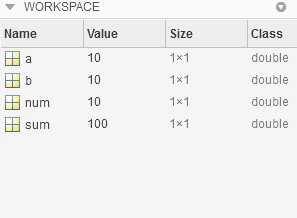Note:

• If you don’t put ‘;’ after the variable name, Matlab will display its content after hitting enter.
• If you don’t give any name to your variable by default Matlab name it with an answer.
• By default, Matlab treats all variables as matrices if you write just 1 it will store it as 1×1 matrix.

To display the content of the Matlab variable you just need to type the name of the variable, and it will show its content on Command-Line:

Example:

## Matlab

 `% MATLAB Code for display variable %``% value in command window %``% First variable 5 `` ``a`` ``a = 100`` ` `% Second variable %`` ``b `` ``b = 200`

Output: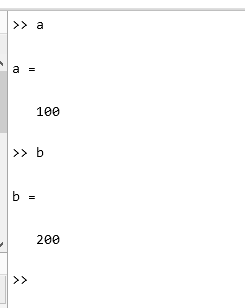### Multiple Assignments of Variables

We can also define the multiple variables in a single line using “;” operator.

Example:

## Matlab

 `% MATLAB code for multiple variable %``% assignment in single line %``x = 12; y = 2; z = x*y; ``disp(``'Result z ='``);``disp(z);`

Output: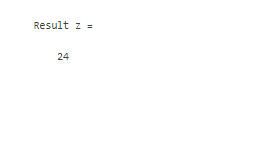### Who command

Who lists in alphabetical order the names of all variables in the currently active workspace. To use this command variables should be present in the active workspace and memory allocated to each variable is necessary.

Example:

## Matlab

 `% MATLAB code for Who command %``x = 12; y = 2; z = x*y; ``disp(``'Result z ='``);``disp(z);`` ` `who`

output: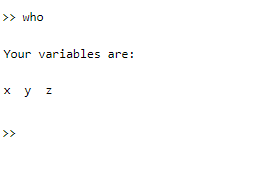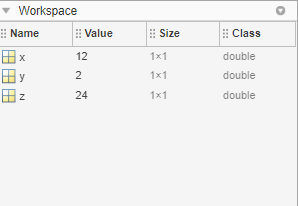### Assigning Vectors to variables

A vector is a one-dimensional array of numbers. Matlab allows you two types of vector:

• Row Vector
• Column Vector.

Row Vectors are created by enclosing numbers in square brackets separating with either space or comma.

Example:

## MATLAB

 `% MATLAB program for row vector %``rowVector = [1 2 3 4 5]`

Output: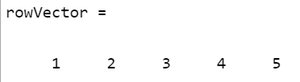Column Vectors are created by enclosing numbers in square brackets, separating each number with ;(semicolon).

Example:

## MATLAB

 `% MATLAB program for column vector % ``columnVector = [1; 2; 3; 4; 5]`

Output: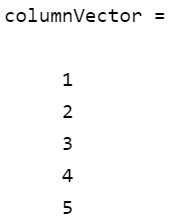### Assigning Matrices to variables

A Matrix is a two-dimensional array of numbers. In Matlab, matrices are created by enclosing numbers in a square bracket write each row of numbers with space or comma separate and after each row put a semicolon. The below code will create a 3×3 matrix:

Example:

## MATLAB

 `% MATLAB program for 3x3 Matrix % ``matalbMatrix = [ 1 2 3; 4 5 6; 7 8 9]`

Output: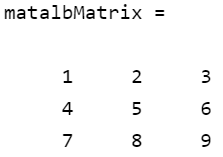My Personal Notes arrow_drop_up